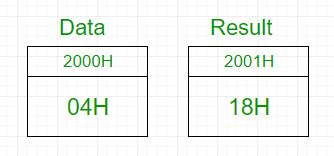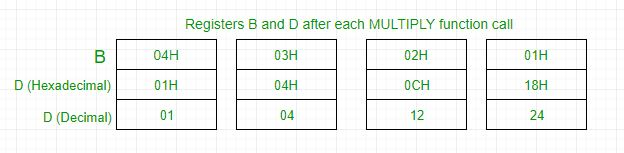GeeksforGeeks App
Open AppBrowser
Continue

# 8085 program to find the factorial of a number

Problem – Write an assembly language program for calculating the factorial of a number using 8085 microprocessor.

Example –

```Input : 04H
Output : 18H
as 04*03*02*01 = 24 in decimal => 18H
```In 8085 microprocessor, no direct instruction exists to multiply two numbers, so multiplication is done by repeated addition as 4×3 is equivalent to 4+4+4 (i.e., 3 times).
Load 04H in D register -> Add 04H 3 times -> D register now contains 0CH -> Add 0CH 2 times -> D register now contains 18H -> Add 18H 1 time -> D register now contains 18H -> Output is 18HAlgorithm –

1. Load the data into register B
2. To start multiplication set D to 01H
4. Decrements B to multiply previous number
6. Take memory pointer to next location and store result
7. Load E with contents of B and clear accumulator
8. Repeatedly add contents of D to accumulator E times
9. Store accumulator content to D
10. Go to step 4
2001HResultResult of factorial
2002HLXI H, 2000HLoad data from memory
2005HMOV B, MLoad data to B register
2006HMVI D, 01HSet D register with 1
2008HFACTORIALCALL MULTIPLYSubroutine call for multiplication
200BHDCR BDecrement B
200CHJNZ FACTORIALCall factorial till B becomes 0
200FHINX HIncrement memory
2010HMOV M, DStore result in memory
2011HHLTHalt
2100HMULTIPLYMOV E, BTransfer contents of B to C
2101HMVI A, 00HClear accumulator to store result